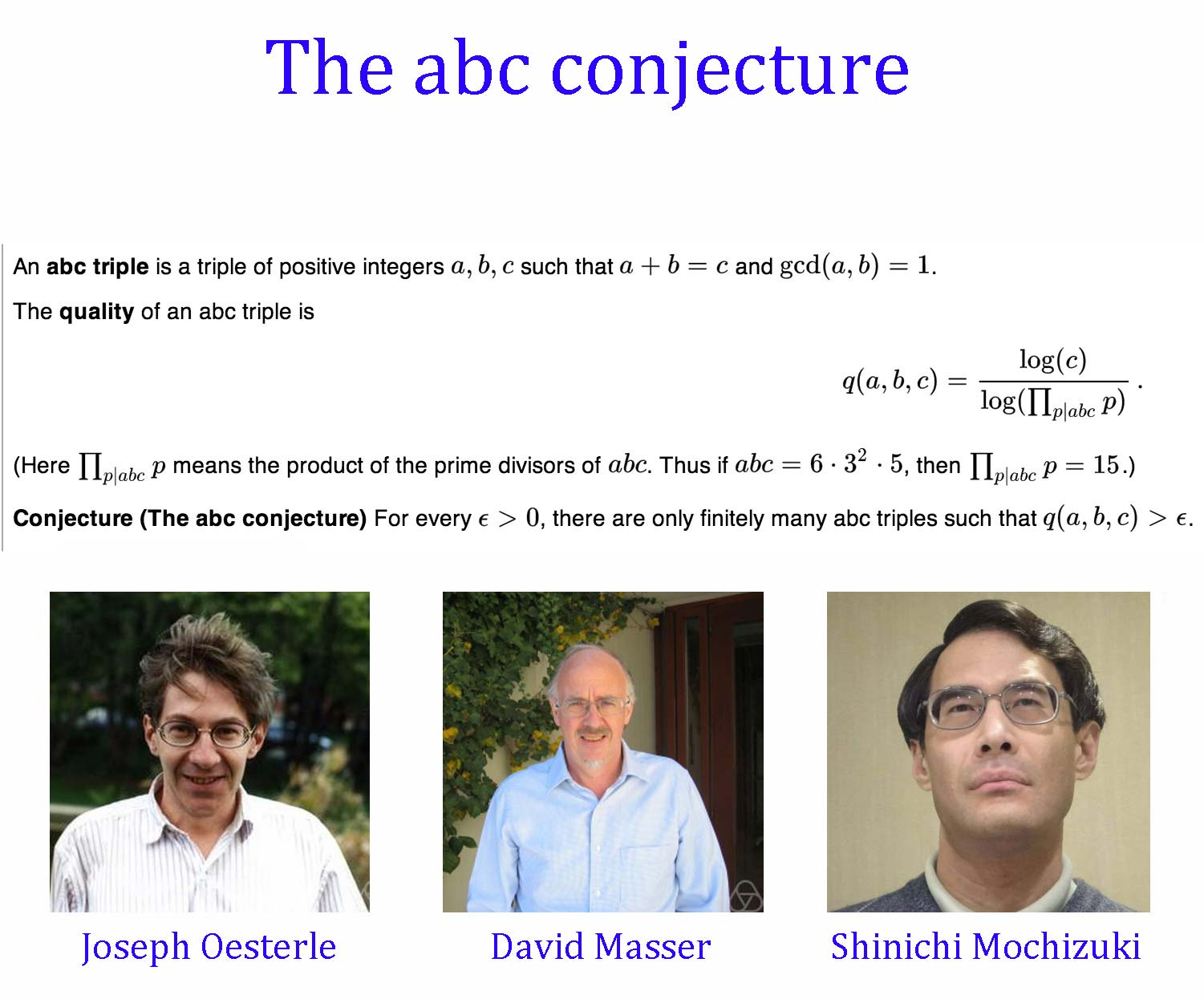A# abc conjectureThe abc conjecture is a remarkable conjecture, first put forward in 1980 by Joseph Oesterle of the University of Paris and David Masser of the Mathematics Institute of the University of Basel in Switzerland, which is now considered one of the most important unsolved problems in number theory (but see the section below this introduction). If it were proved correct, the proofs of many other famous conjectures and theorems would follow immediately – in some cases in just a few lines. The vastly complex current proof of Fermat's last theorem, for example, would reduce to less than a page of mathematical reasoning. The ABC conjecture is disarmingly simple compared to most of the deep questions in number theory and, moreover, turns out to be equivalent to all the main problems that involve Diophantine equations (equations with integer coefficients and integer solutions).

Only a couple of concepts need to be understood to grasp the abc conjecture. A square-free number is an integer that isn't divisible by the square of any number except 1. For example, 15 and 17 are square-free, but 16 (divisible by 42) and 18 (divisible by 32) are not. The square-free part of an integer n, denoted sqp(n), is the largest square-free number that can be formed by multiplying the prime factors of n. Thus, for n = 15, the prime factors are 5 and 3, and 3 × 5 = 15, a square-free number. So sqp(15) = 15. On the other hand, for n = 16, the prime factors are all 2, which means that sqp(16) = 2. Similarly, sqp(17) = 17 and sqp(18) = 6. In general, if n is square-free, the square-free part of n is just n; otherwise, sqp(n) represents what is left over after all the factors that create a square have been eliminated. In other words, sqp(n) is the product of the distinct prime numbers that divide n. For example, sqp(9) = sqp(3 × 3) = 3 and sqp(1400) = sqp(2 × 2 × 2 × 5 × 5 × 7) = 2 × 5 × 7 = 70.

The abc conjecture deals with pairs of numbers that have no common factors. Suppose a and b are two such numbers and that c is their sum. For example, if a = 3 and b = 7, then c = 3 + 7 = 10. Now, consider the square-free part of the product a × b × c: sqp(abc) = sqp(3 × 7 × 10) = 210. For most choices of a and b, sqp(abc) is greater than c, as in the example above. In other words, sqp(abc)/c > 1. Occasionally, however, this isn't true. For instance, if a = 1 and b = 8, then c = 1 + 8 = 9, sqp(abc) = sqp(1 × 8 × 9) = sqp(1 × 2 × 2 × 2 × 3 × 3) = 1 × 2 × 3 = 6, and sqp(abc)/c = 6/9 = 2/3. Similarly, if a = 3 and b = 125, the ratio is 15/64, and if a = 1 and b = 512, the ratio is 2/9.

David Masser proved that the ratio sqp(abc)/c can get arbitrarily small. In other words, given any number greater than zero, no matter how small, it's possible to find integers a and b for which sqp(abc)/c is smaller than this number. In contrast, the abc conjecture states that [sqp(abc)]n/c does reach a minimum value if n is any number greater than 1 – even a number such as 1.0000000000001, which is just barely larger than 1. The tiny change in the expression makes a vast difference in its mathematical behavior. The abc conjecture in effect translates an infinite number of Diophantine equations (including the equation of Fermat's last theorem) into a single mathematical statement.

## The abc conjecture proved?

In 2012, Shin Mochizuki claimed to have proved the abc conjecture using a new set of techniques, which he calls "inter-universal geometry" – a generalization of the foundations of algebraic geometry. In a nutshell, Mochizuki has devised a new scheme of mathematical objects, which he believes he understands well enough to apply to proving this long-standing problem in Diophantine analysis.

It will take some time for other mathematicians to check through Mochizuki's proof because first they will have to make themselves familiar with the world of inter-universal geometry. But Mochizuki reputation is sufficiently high, and the conjecture sufficiently important, that the effort will be made.

### Reference

1. Guy, Richard K. Unsolved Problems in Number Theory, 2nd ed. New York: Springer-Verlag, 1994.